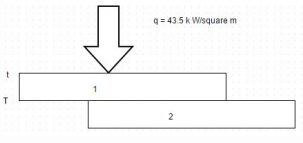Courses

# Test: General Heat Conduction Equation

## 10 Questions MCQ Test Heat Transfer | Test: General Heat Conduction Equation

Description
This mock test of Test: General Heat Conduction Equation for Chemical Engineering helps you for every Chemical Engineering entrance exam. This contains 10 Multiple Choice Questions for Chemical Engineering Test: General Heat Conduction Equation (mcq) to study with solutions a complete question bank. The solved questions answers in this Test: General Heat Conduction Equation quiz give you a good mix of easy questions and tough questions. Chemical Engineering students definitely take this Test: General Heat Conduction Equation exercise for a better result in the exam. You can find other Test: General Heat Conduction Equation extra questions, long questions & short questions for Chemical Engineering on EduRev as well by searching above.
QUESTION: 1

### From the concept of kinetic theory, mean travel velocity of the gas molecules is prescribed by the relation Where, G = Universal gas constant M = Molecular weight of the gas T = Absolute temperature

Solution:

Heat transfer by conduction in gases occurs through transport of the kinetic energy of molecular motion resulting from the random movement and collisions of the molecules.

QUESTION: 2

### Low temperature insulation are used when the enclosure is at a temperature lower than the ambient temperature. Which one of the following is not a low temperature insulation?

Solution:

Asbestos is a high temperature insulation which are used when it is desired to prevent an enclosure at a temperature higher than the ambient from losing heat to its surroundings.

QUESTION: 3

### The value of Lorenz number in 10-8 W ohms/K2 is

Solution:

Lorenz no. = k/α T

QUESTION: 4

For liquids, thermal conductivity is governed by the relation

Where,
C= Specific heat at constant pressure
M = Molecular weight of the gas
p = Mass density
A = Area

Solution:

Area doesn’t depends on the nature of liquid but on the quantity (Ac p) is nearly constant for all liquids.

QUESTION: 5

Consider the following parameters
(i) Composition
(ii) Density
(iii) Porosity
(iv) Structure
Then, thermal conductivity of glass wool varies from sample to sample because of variation is

Solution:

There is a variation due to all above options.

QUESTION: 6

The thermal conductivity and the electrical conductivity of a metal at absolute temperature are related as

Solution:

It is defined as the ratio of thermal conductivity to the product of electrical conductivity and temperature.

QUESTION: 7

The relation Ϫ2 t =0 is referred to as

Solution:

In the absence of internal heat generation or release of energy within the body, equation reduces to Laplace equation.

QUESTION: 8

The unit of thermal diffusivity is

Solution:

The quantity α = k/pc is called thermal diffusivity.

QUESTION: 9

To effect a bond between two metal plates, 2.5 cm and 15 cm thick, heat is uniformly applied through the thinner plate by a radiant heat source. The bonding must be held at 320 K for a short time. When the heat source is adjusted to have a steady value of 43.5 k W/m2, a thermocouple installed on the side of the thinner plate next to source indicates a temperature of 345 K. Calculate the temperature gradient for heat conduction through thinner plate. In the diagram, the upper plate is 2.5 cm thick while the lower is 15 cm thick.

Solution:Temperature gradient = d t/d x = – 1000 degree Celsius/m. Also, q/A = – k (t2– t 1)/δ.

QUESTION: 10

The diffusion equations
Ɏ2t + q = (1/α) (d t/d r)
Governs the temperature distribution under unsteady heat flow through a homogenous and isotropic material. The Fourier equation follows from this expression when

Solution:

In unsteady state condition, there is no internal heat generation.